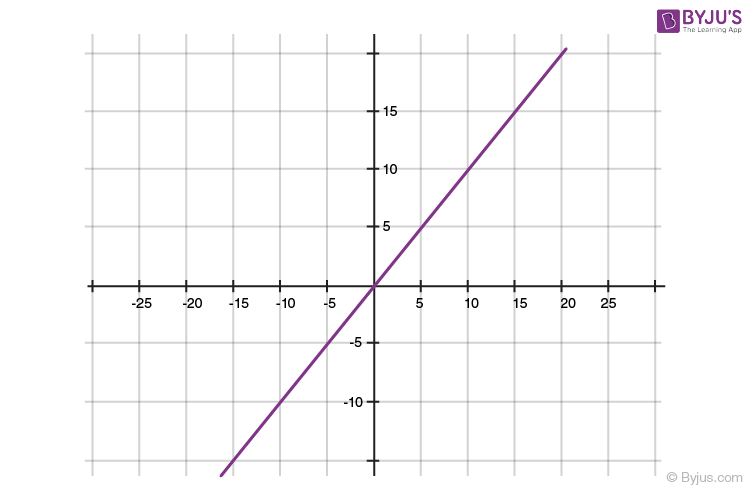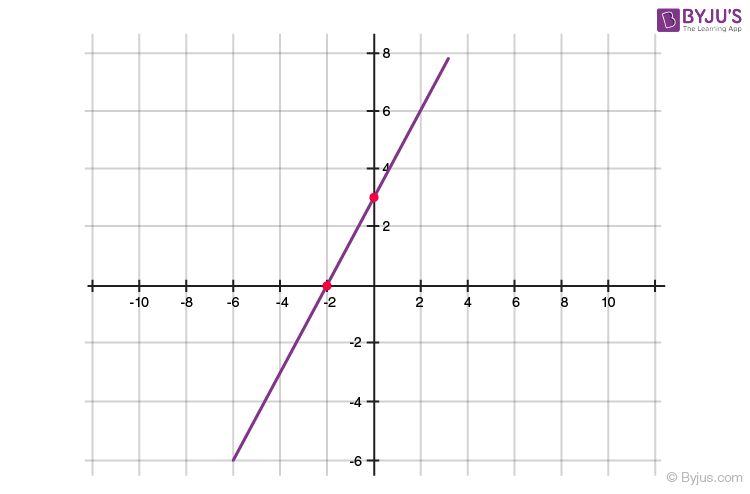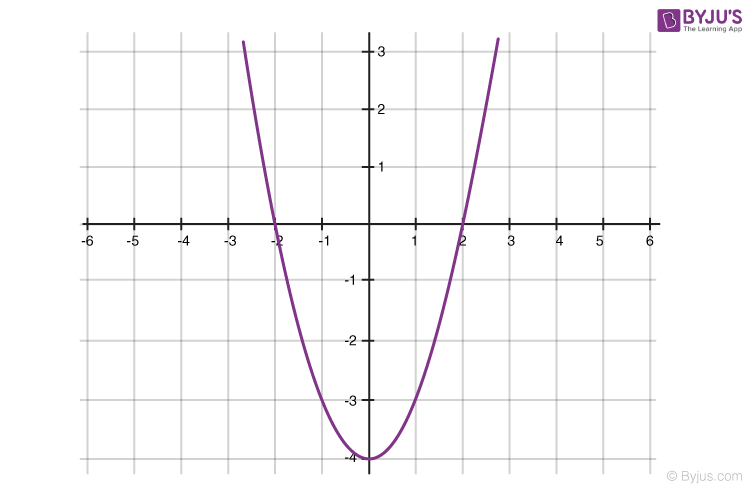# Polynomial Class 10 Notes: Chapter 2

## CBSE Class 10 Maths Notes Polynomial:-

Polynomials for class 10 concepts are given in detail. Go through the below article to learn the concept of polynomials such as expressions, degrees, types, graphical representation and so on.

## Algebraic Expressions

An algebraic expression is an expression made up of variables and constants along with mathematical operators.

An algebraic expression is a sum of terms, which are considered to be building blocks for expressions.

A term is a product of variables and constants. A term can be an algebraic expression in itself.
Examples of a term – 3 which is just a constant.
– 2x, which is the product of constant ‘2’ and the variable ‘x’
– 4xy, which is the product of the constant ‘4’ and the variables ‘x’ and ‘y’.
– 5x2y, which is the product of 5, x, x and y.

The constant in each term is referred to as the coefficient.

Example of an algebraic expression: 3x2y+4xy+5x+6 which is the sum of four terms: 3x2y, 4xy, 5x and 6.

An algebraic expression can have any number of terms. The coefficient in each term can be any real number. There can be any number of variables in an algebraic expression. The exponent on the variables, however, must be rational numbers.

To know more about Algebraic Expressions, visit here.

### Polynomial

An algebraic expression can have exponents that are rational numbers. However, a polynomial is an algebraic expression in which the exponent on any variable is a whole number.

5x3+3x+1 is an example of a polynomial. It is an algebraic expression as well.

2x+3√x is an algebraic expression, but not a polynomial. – since the exponent on x is 1/2 which is not a whole number.

To know more about Polynomial, visit here.

### Degree of a Polynomial

For a polynomial in one variable – the highest exponent on the variable in a polynomial is the degree of the polynomial.

Example: The degree of the polynomial x2+2x+3 is 2, as the highest power of x in the given expression is x2.

## Types Of Polynomials

Polynomials can be classified based on:
a) Number of terms
b) Degree of the polynomial.

### Types of polynomials based on the number of terms

a) Monomial – A polynomial with just one term. Example: 2x, 6x2, 9xy

b) Binomial – A polynomial with two terms. Example: 4x2+x, 5x+4

a) Trinomial – A polynomial with three terms. Example: x2+3x+4

### Types of Polynomials based on Degree

Linear Polynomial

A polynomial whose degree is one is called a linear polynomial.
For example, 2x+1 is a linear polynomial.

A polynomial of degree two is called a quadratic polynomial.
For example, 3x2+8x+5 is a quadratic polynomial.Cubic Polynomial

A polynomial of degree three is called a cubic polynomial.
For example, 2x3+5x2+9x+15 is a cubic polynomial.

## Graphical Representations

Let us learn here how to represent polynomial equation on the graph.

### Representing Equations on a Graph

Any equation can be represented as a graph on the Cartesian plane, where each point on the graph represents the x and y coordinates of the point that satisfies the equation. An equation can be seen as a constraint placed on the x and y coordinates of a point, and any point that satisfies that constraint will lie on the curve

For example, the equation y = x, on a graph, will be a straight line that joins all the points which have their x coordinate equal to their y coordinate. Example – (1,1), (2,2) and so on.### Geometrical Representation of a Linear Polynomial

The graph of a linear polynomial is a straight line. It cuts the X-axis at exactly one point.### Geometrical Representation of a Quadratic Polynomial

• The graph of a quadratic polynomial is a parabola
• It looks like a U which either opens upwards or opens downwards depending on the value of ‘a’ in ax2+bx+c
• If ‘a’ is positive, then parabola opens upwards and if ‘a’ is negative then it opens downwards
• It can cut the x-axis at 0, 1 or two points
•Test your knowledge on Polynomial For Class 10

1. B Hema Shalini

it was very useful

2. This notes helped me a lot.

Very helpful and best material ever!!!!!!!!!!!1111